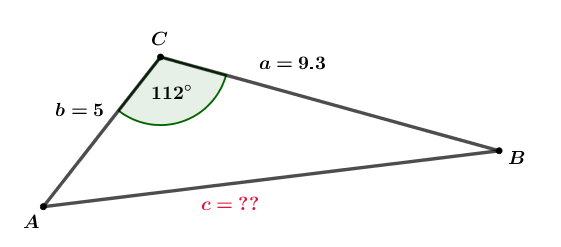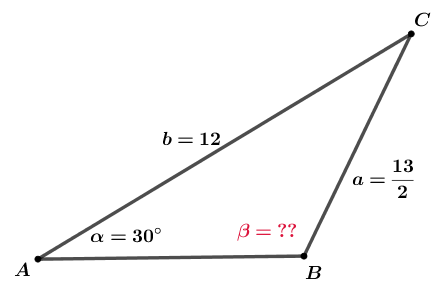Math Calculators, Lessons and Formulas

It is time to solve your math problem

mathportal.org

# Triangle calculator

This calculator applies the Law of Sines $~~ \dfrac{\sin\alpha}{a} = \dfrac{\cos\beta}{b} = \dfrac{cos\gamma}{c}~~$ and the Law of Cosines $~~ c^2 = a^2 + b^2 - 2ab \cos\gamma ~~$ to solve oblique triangles, i.e., to find missing angles and sides if you know any three of them.

The calculator shows all the steps and gives a detailed explanation for each step.

Oblique Triangle Calculator
input three values and select what to find
show help ↓↓ examples ↓↓
The missing value is:
Input three elements of a triangle:
calculator works with decimals, fractions and square roots (to input $\color{blue}{\sqrt{2}}$ type $\color{blue}{\text{r2}}$)
$a$
=
$b$
=
$c$
=
$\alpha$
=
$\beta$
=
$\gamma$
=
Area
=
working...
examples
example 1:ex 1:
A triangle has sides equal to $3 m$, $5 m$ and $6 m$. Find angle $\alpha$.
example 2:ex 2:
What is the length of side c?example 3:ex 3:
Find angle $\beta$.Search our database of more than 200 calculators